Yu, X., Chen, C., Demirel, S., and Zhang, J. (2019). "Static lateral load resistance of parallel bamboo strand panel-to-metal single-bolt connections – Part 2: Fracture model," BioRes. 14(3), 5747-5763.

#### Abstract

The effect of end distance was evaluated relative to the static ultimate lateral resistance load of single-shear unconstrained metal-to-parallel bamboo strand panel (PBSP) single-bolt connections that failed in end tear-out, net cross-section tension modes. Empirical equations for estimation of the static ultimate lateral resistance loads of the evaluated connections were derived. The connection consisted of a PBSP main member fastened to a metal plate as a side member using a 6 mm diameter bolt without a nut or washer. The stress concentration factor was considered in the process of deriving the estimation equations for static ultimate lateral resistance loads. The experimental results indicated that the stress concentration factor was a linear function of end-distance to bolt-diameter ratio, (e/d), for connections that failed in end tear-out mode, while a quadratic function of (e/d) for connections that failed in net cross-section tension mode. The derived estimation equations including stress concentration factor can reasonably estimate the static ultimate lateral resistance load of single-shear unconstrained metal-to-PBSP single-bolt connections evaluated in this study.

Static Lateral Load Resistance of Parallel Bamboo Strand Panel-to-Metal Single-Bolt Connections – Part 2: Fracture Model

Xiaohong Yu,a,* Chen Chen,b,c,* Samet Demirel,d and Jilei Zhang b

The effect of end distance was evaluated relative to the static ultimate lateral resistance load of single-shear unconstrained metal-to-parallel bamboo strand panel (PBSP) single-bolt connections that failed in end tear-out, net cross-section tension modes. Empirical equations for estimation of the static ultimate lateral resistance loads of the evaluated connections were derived. The connection consisted of a PBSP main member fastened to a metal plate as a side member using a 6 mm diameter bolt without a nut or washer. The stress concentration factor was considered in the process of deriving the estimation equations for static ultimate lateral resistance loads. The experimental results indicated that the stress concentration factor was a linear function of end-distance to bolt-diameter ratio, (e/d), for connections that failed in end tear-out mode, while a quadratic function of (e/d) for connections that failed in net cross-section tension mode. The derived estimation equations including stress concentration factor can reasonably estimate the static ultimate lateral resistance load of single-shear unconstrained metal-to-PBSP single-bolt connections evaluated in this study.

Keywords: Bolt connections; Bamboo panels; Static load; Shear strength; Tensile strength; Stress concentration factor

Contact information: a: School of Engineering, Zhejiang Agriculture and Forestry University, Zhejiang, China; b: Department of Sustainable Bioproducts, Mississippi State University, Mississippi State, MS 39762-9820; c: College of Furnishings and Industrial Design Nanjing Forestry University Nanjing, Jiangsu, China; d: Department of Forest Industrial Engineering Faculty of Forestry Karadeniz Technical University Trabzon, Turkey 61080; * Corresponding authors: yuxiaohong@zafu.edu.cn and cc3686@msstate.edu

INTRODUCTION

Parallel bamboo strand panels (PBSPs) are a mixture of thermally treated bamboo strands with thermoset adhesives such as phenol-formaldehyde. The mixture mat can be hot pressed to form PBSPs. In recent years, PBSPs have shown tremendous growth in the flooring market, and many flooring companies are interested in potential applications of PBSPs as frame stocks for outdoor furniture.

A single-shear metal-to-PBSP single-bolt connection is commonly used in jointing PBSP structural components in outdoor furniture constructions such as park benches because it provides an effective and convenient method for furniture installation, dismantling, and repair. Knowing the static lateral load resistance capacity of a single-shear metal-to-PBSP single-bolt connection is therefore important for strength and safety design of outdoor furniture frames in PBSPs.

Common failure modes of a single-shear metal-to-wood or wood-based composites single-bolt connection when subjected to a static lateral load could include four different types: yield, tear-out, splitting, and net cross-section, as shown in Fig. 1 (Wang et al. 2017).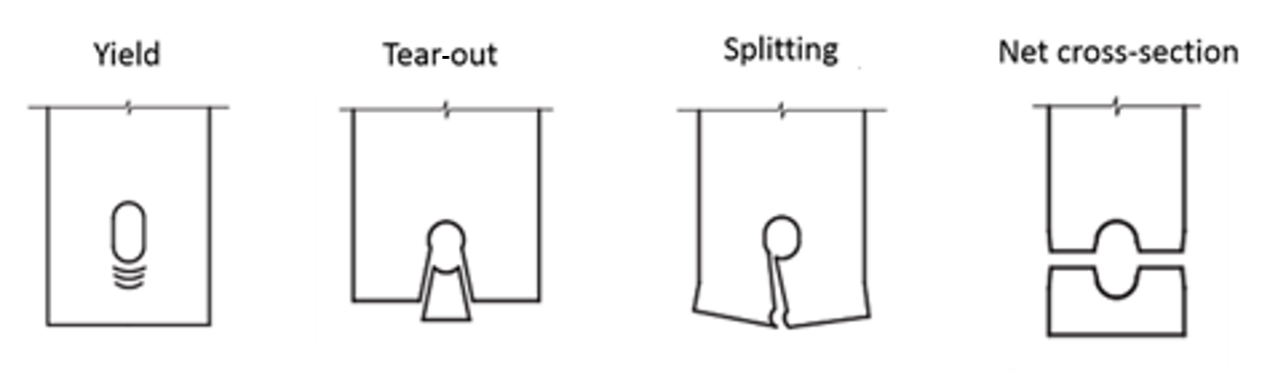Fig. 1. Four types of failure modes commonly seen in a single-shear metal-to-wood or wood-based composite single-bolt connection

In general, the prediction equations of the static lateral resistance load of a single-shear metal-to-wood (Wilkinson 1978; Blass et al. 1995; ANSI/AWC NDS-2012 2012) or wood-based composite (Kuang et al. 2017; Yu et al. 2017) single-bolt connection that can fail with yield modes are derived based on the European Yield Model of Johansen (1949). Fundamentally, a mechanics-based approach was used to derive the equations with the consideration of bolt moment resistance and bolt-bearing resistance in connection main member materials as main factors. In the case of a connection that can fail in fracture modes such as tear-out, splitting, and net cross-section, the stress distribution (i.e., stress concentration) that occurs at the shear section or tension cross-section caused by the bolt hole is very complex at the point where the connection static lateral resistance load capacity reaches its ultimate value. The main factors considered regarding the lateral resistance load of a single-shear bolt connection that can fail in fracture modes can include strength properties of connection member materials such as shear, tensile strengths, and member geometry including hole-diameter, bolt-diameter, etc.

Wang et al. (2017) investigated the effects of end distance, edge distance, and steel grade on the static lateral resistance load of single-shear one-bolt high strength steel connections that failed in fracture modes. They derived prediction equations for lateral resistance loads based on Eurocode 3 (CEN 2005, EN 1993-1-8 2005, EN-1993-1-1 2005, EN 1993-1-12 2007) and ANSI/AISC 360-10 (2010) methods and concluded that equations derived based on the Eurocode 3 method could conservatively predict the static lateral resistance load of single-shear single-bolt steel connections in high strength steels with their nominal tensile strength up to 890 MPa. The equations based on the ANSI/AISC 360-10 (2010) method tend to overestimate the static lateral resistance load of single-shear single-bolt steel connections.

Eurocode 3 and ANSI/AISC 360-10 (2010) methods are typical regression analysis approaches commonly performed in steel materials based on testing data to derive prediction equations for the static lateral resistance load of bolt connections in normal and high strength steel plates. The common equation (Eq. 1) used in Eurocode 3 to calculate the maximum lateral load is,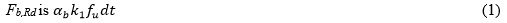where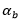and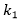are the stress concentration factors related to end distance and edge distance, i.e. (Eqs. 2 and 3),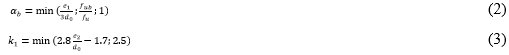where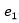and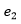are end distance and edge distance of a tested connection, respectively;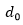is the bolt diameter;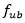and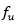are ultimate strengths of the bolt and member material. The ANSI/AISC 360-10 (2010) method is different from the Eurocode 3 one, i.e. when the deformation around the bolt hole is not a design consideration at a service load, the bearing resistance at the bolt hole,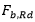, is calculated using Eq. 4,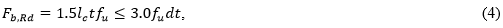where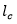is the clear distance in the direction of the force acting between the edge of the hole and the edge of the adjacent hole or edge of the material. Moze and Beg (2010) proposed an improved model of Eurocode 3 using stress concentration factors, where Eqs. 5 and 6 replace Eq. 3.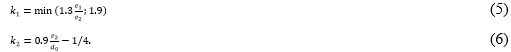Kim and Yura (1999) investigated the effects of end distance, spacing, and steel strength on the bearing strength of one-bolt and two-bolt lap connections and concluded that the stress concentration factor is a fixed value because the results of the stress ratio calculated using the Eurocode method is the same as the experimental results. Duerr (2006) presented an experiment evaluating the strength of pinned connections through focusing on three failure modes of tear-out, splitting, and net cross-section and concluded that the stress concentration factor was an important component in a predictive model. A stress concentration on ultimate tension strength was considered in the final ultimate point load equation. The applied load on the ultimate point P is calculated using Eq. 7,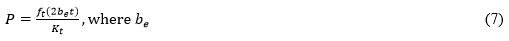where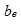is the edge distance; is the thickness of a tested specimen; and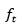is the maximum tension stress (MPa). The parameter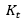is the stress concentration factor calculated using the Eq. 8: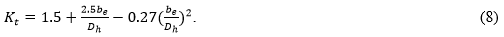He et al. (2016) developed an experimental strategy for estimating the strength of single-shear metal bolt connections together with the finite element model method verifying the calculation. The average stress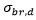is calculated using Eq. 9,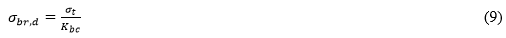where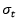is the maximum stress; and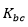is the stress concentration factor determined using the following Eqs. 10 and 11: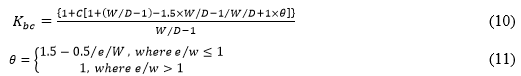where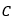is the stress concentration mitigation factor based on material type,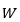is the width distance of a tested specimen,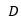is the bolt diameter, and is the edge distance.

Limited literature was found in evaluating and modeling the lateral resistance load of single-shear metal-to-PBSP single-bolt connection that failed in fracture modes of connection members, especially with a bolt diameter equal to or less than 6 mm, which were the common sizes used in outdoor furniture construction. A series of studies (Yu et al. 2017) were performed to investigate major factors such as end distance, PBSP shear, tensile strengths, and bolt-bearing strengths in PBSP materials on the lateral load resistance behavior of single-shear unconstrained metal-to-PBSP single-bolt connections in outdoor sitting furniture applications. Yu et al. (2017) derived the prediction equations of the static lateral resistance load of a single-shear metal-to- PBSP single-bolt based on the European Yield Model of Johansen (1949) for those connections that failed with yield modes, and even though the lateral resistance load of single-shear metal-to-PBSP single-bolt that failed in fracture modes of connection members were evaluated and reported, but no prediction equations were derived.

This article reports results mainly from the investigation of single-shear unconstrained metal-to-PBSP single-bolt connections that failed in fracture modes such as end tear-out and net cross-section tension. Specific objectives of this study were 1) to evaluate the tensile strength of PBSP materials perpendicular to bamboo strand orientation; 2) to evaluate the shear strength of PBSP materials parallel to bamboo strand orientation; 3) to evaluate the effect of end distance on the static lateral resistance load of single-shear unconstrained metal-to-PBSP single-bolt connections that failed in end tear-out mode; 4) to evaluate the effect of end distance on the static lateral resistance load of single-shear unconstrained metal-to-PBSP single-bolt connections that failed in net cross-section tension mode; and 5) to derive estimation equations for the static lateral resistance load of single-shear unconstrained metal-to-PBSP single-bolt connections.

EXPERIMENTAL

Materials

Full-sized PBSPs provided by Hangzhou Dasuo Technology Co., Ltd, Zhejiang, China measuring 1800 mm long × 1200 mm wide × 20 mm thick were used in this experiment. Bamboo strands of PBSPs were oriented in the direction parallel to panel length direction and hot-pressed in the direction parallel to its thickness direction. The species of bamboo strands is Phyllostachys heterocycla (Carr.) Mitford cv. pubescens, and the origin of this bamboo is the Zhejiang province. The 6 mm × 50 mm, C Grade, Q235 carbon steel hex bolts were obtained from a location hardware store (Linan, Zhejiang, China) (GB/T 5780-200 2000; ASTM F1575-03 2003; ASTM D5764-97a 2018). The 8 mm thick metal plate material was regular carbon steel.

Experimental Design

Bolt connections

The general configuration of a single-shear unconstrained metal-to-PBSP single-bolt connection is shown in Fig 2a. The unconstrained connection consisted of a PBSP main member attached to a metal side member through a single bolt without a nut or washer used. The metal side member measured 223 mm long × 51.8 mm wide × 8 mm thick. A Grade, Q235 carbon steel.

A complete 3 × 2 factorial experiment with 4 replications per combination was conducted to derive and validate the estimation equation for the static lateral resistance load of single-shear unconstrained metal-to-PBSP single-bolt connections subjected to lateral loads parallel to strand orientation of main members. The two factors were end distance, e (12 mm, 18 mm, and 24 mm, which were 2, 3, and 4 times of the bolt diameter, d, of 6 mm, respectively) and edge distance (18 mm and 30 mm, which were 3 and 5 times of the bolt diameter of 6 mm, respectively) as shown in Fig. 2b. The edge distance 18 mm data set was used to derive the estimation equation, while the edge distance 30 mm data set was used to validate the derived estimation equation.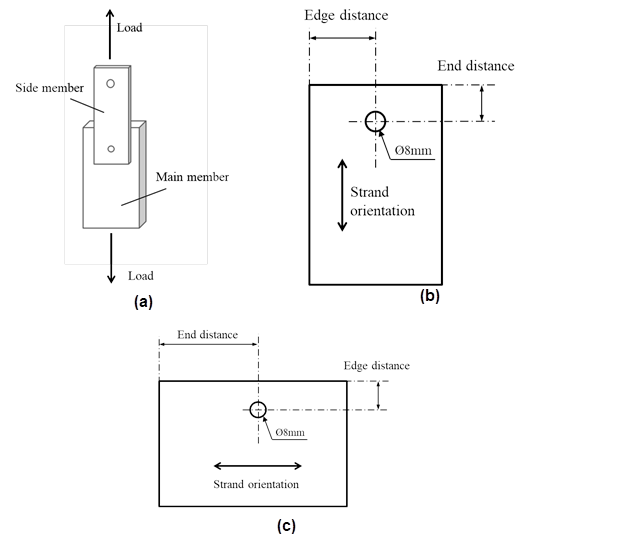Fig. 2. The conﬁguration of a single-shear unconstrained metal-to-PBSP single-bolt connection: (a) detailed dimensions of PBSP main member parallel, (b) perpendicular, and (c) to bamboo strand orientation

A complete 6 × 2 factorial experiment with 4 replications per combination was conducted to derive and validate the estimation equation for the static lateral resistance load of single-shear unconstrained metal-to-PBSP single-bolt connection subjected to lateral loads perpendicular to strand orientation of main members. The two factors were end distance, e (36, 48, 60, 66, 72, and 78 mm, which were 6, 8, 10, 11, 12, and 13 times of the bolt diameter, d, of 6 mm, respectively) and edge distance (24 mm and 36 mm, which were 4 and 6 times the bolt diameter of 6 mm, respectively) as shown in Fig. 2c. The edge distance 24 mm data set was used to derive the estimation equation, while the edge distance 36 mm data set was used to validate the derived estimation equation.

Basic material properties

Tensile properties of 36 PBSP specimens perpendicular to the bamboo strand orientation were tested according to GB 1938-2009 (2009a), and Fig. 3a shows the configuration and detailed dimension of a tensile test specimen. Shear strength on the surface parallel to thickness surface of 36 PBSP specimens parallel to bamboo strand orientation was evaluated according to GB 1937-2009 (2009b), and Fig. 3b shows the configuration and detailed dimensions of a shear test specimen. Specific gravity and moisture content of PBSPs were tested accordingly to GB 1933-2009 (2009c) and GB 1931-2009 (2009d), respectively.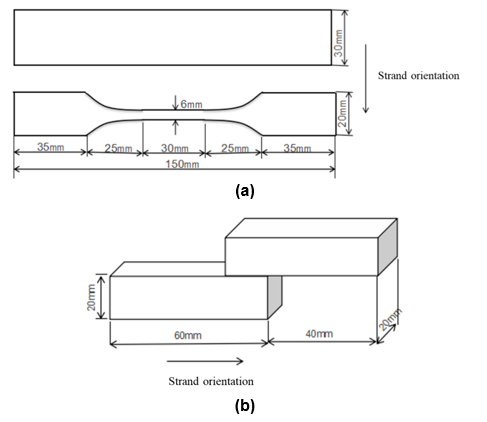Fig. 3. General configurations of tensile (a) and shear (b) strength specimens

Specimen Preparation and Testing

Four full-sized PBSPs (1800 mm long × 1200 mm wide × 20 mm thick) were used in this study. Figure 4a shows the cutting pattern for each of the four panels, and Fig. 4b shows how physical and mechanical property specimens were cut from the precut 400 mm × 300 mm panels. All connection main members were randomly selected from the connection specimen supply prepared from cutting full-size PBSPs based on the cutting pattern. Before testing, all specimens were conditioned in a humidity chamber controlled at 20 °C ± 2 °C and 65% ± 5% RH for 40 h.

All tensile, shear, and connection tests were performed on a DNS50 universal testing machine (Changchun Machinery Research Institute, Changchun, China). Figure 5 shows the setups for evaluating tensile (a) and shear (b) strengths of PBSP materials.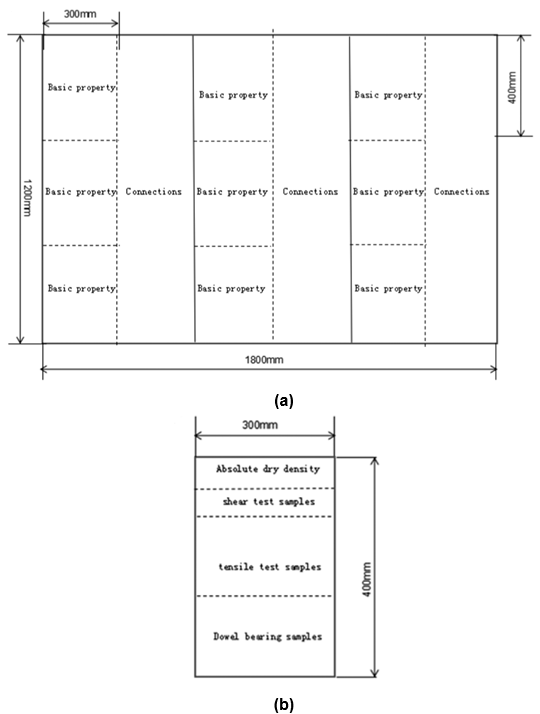Fig. 4. Cutting patterns showing (a) where parallel strand bamboo panel (PBSP) mechanical property and connection specimens were cut from full-size PBSPs, and (b) how PBSP mechanical property specimens were cut

Figure 6 shows the setup for evaluating lateral resistance loads of single-shear unconstrained metal-to-PBSP single-bolt connections. The loading speed was 3 mm/min (ASTM D5652-95 2007). Ultimate loads and failure modes of all tested connections were recorded.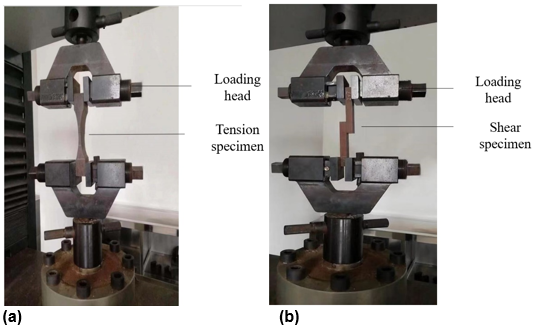Fig. 5. Setups for evaluating the tensile (a) and shear (b) strengths of PBSP materials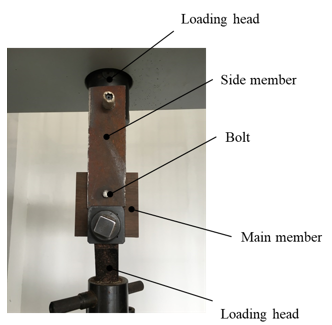Fig. 6. Setup for evaluating the lateral resistance load of single-shear unconstrained metal-to-PBSP single-bolt connections

RESULTS AND DISCUSSION

Basic Material Properties

The PBSP specific gravity averaged 1.09 with a coefficient of variation (COV) of 6.4%, whereas the PBSP MC averaged 8.55% with a COV of 7.0% based on 36 replicates. The PBSP tensile strength perpendicular to bamboo strand orientation of 36 replicates, σult, averaged 7.26 MPa with a COV of 11.8%. PBSP shear strength parallel to bamboo strand orientation of 36 replicates, τult, averaged 11.04 MPa with a COV of 15.6%.

Bolt Connections

Failure modes

Figure 7 shows three types of failure modes that occurred at PBSP main members together with the bolt bent or not. Type I was end tear-out, i.e. the end of the PBSP main member sheared off in bamboo strand orientation (Fig. 7a) without the bolt bent, and this failure mode occurred only in the connections with their main members subjected to lateral loads parallel to bamboo strand orientation.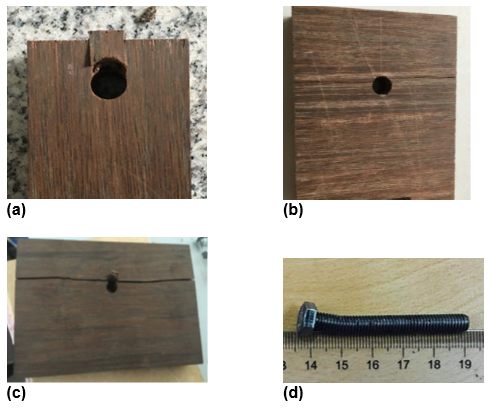Fig. 7. Typical failure modes observed in testing single-shear metal-to-PBSP single-bolt connections under lateral loads: (a) end tear-out; (b) net cross-section tension; (c) bamboo material beneath the bolt compressively fractured; and (d) one-point bent bolt

Type II was net cross-section tension only, i.e. the PBSP main member split along bamboo strand orientation (Fig. 7b) without the bolt becoming bent, and this failure mode happened in the connections with their main members subjected to lateral loads perpendicular to bamboo strand orientation and having end distance less than 10 d (60 mm). Type III was bamboo material beneath the bolt compressively fractured and split along bamboo strand orientation (Fig. 7c) with a one-point bent bolt (Fig. 7d), and this failure mode happened in the connections with their main members subjected to lateral loads perpendicular to bamboo strand orientation and having end distance greater than 10 d (60 mm). Table 1 summarizes failure modes of connections evaluated in this study by subjecting them to lateral loads parallel and perpendicular to bamboo strand orientation.

Table 1. Summary of Failure Mode and Mean Ultimate Lateral Resistance Loads of Single-Shear Metal-to-PBSP Single-Bolt Connection Sets Evaluated in this Study for the Derivation of Estimation Equations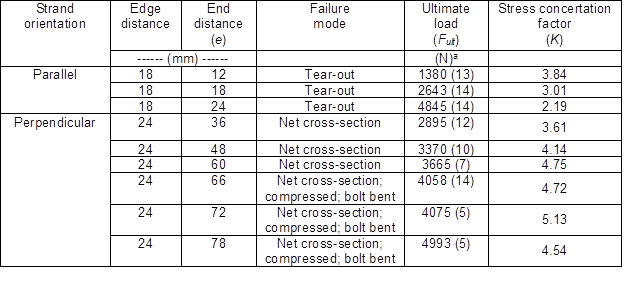a Value in parentheses are coefficients of variation in percentage.

Prediction on ultimate lateral resistance loads

The mean values of ultimate lateral resistance loads of single-shear metal-to-PBSP single-bolt connections tested for deriving estimation equations are summarized in Table 1. The ultimate lateral resistance load, Fult, is expressed as Fult-s when connections failed in end tear-out mode, and Fult-t when connections failed in net cross-section tension mode.

The major failure modes (Table 1) observed in single-shear metal-to-PBSP single-bolt connections were tear-out and net cross-section, indicating that the ultimate lateral resistance load of single-shear metal-to-PBSP single-bolt connections was mainly governed by the shear strength parallel to its strand orientation and tensile strength perpendicular to its strand orientation of PBSP materials. Eurocode 3 and ANSI/AISC 360-10 (2010) methods, i.e., using the regression analysis approaches to finding the relationship between the stress concentration factor and edge or end distance of a connection main member, was proposed to derive the prediction equation for the ultimate lateral resistance load of single-shear metal-to-PBSP single-bolt connections in this study. This was because the stress distribution (i.e., stress concentration) occurred at the shear section or tension cross-section caused by the bolt hole is very complex at the point where the connection lateral resistance load capacity reaches its ultimate value.

The mean values (Table 1) of stress concentration factor, K, of connections failed in the modes of end tear-out and cross-section tension, are plotted as a function of (e/d) in Fig. 8. The stress concentration factor is expressed as Ks when connections failed in end tear-out mode in Eq. 12,

Ks = Fult-s/2teτult (12)

and Kt when connections failed in cross-section tension mode in Eq. 13,

Kt = Fult-t/2teσult (13)

where is the main member thickness (mm), e is the end distance (mm).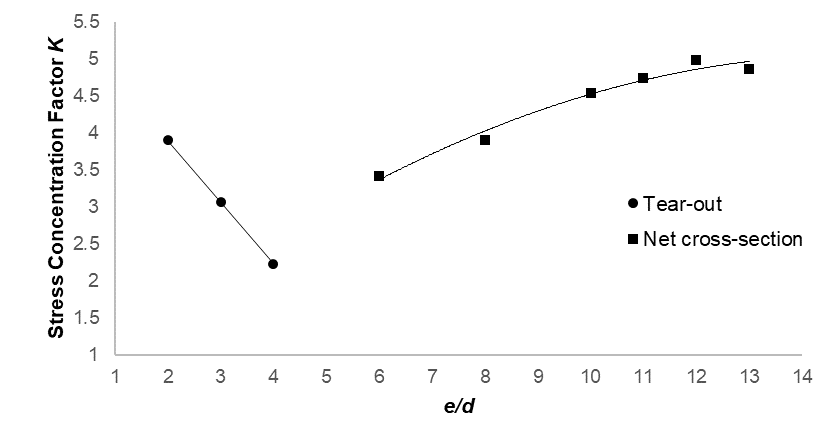Fig. 8. Mean values of stress concentration factor, K, plotted as a function of end-distance to bolt-dimeter ratio, (e/d), for derivation data sets of single-shear metal-to-PBSP single-bolt connections failed in end tear-out and cross-section tension modes

The Eurocode 3 and ANSI/AISC 360-10 (2010) methods were proposed in this study to obtain functional relationships between the stress concentration factor and end-distance to bolt-diameter ratio, (e/d), that might be practical for deriving estimation equations for ultimate lateral resistance loads of single-shear metal-to-PBSP single-bolt connections failed in end tear-out and cross-section tension modes.

Figure 8 indicates that a linear relationship existed between the stress concentration factor and end-distance to bolt-diameter ratio of connections that failed in end tear-out mode. Therefore, a linear function by Eq. 14,

Ks =a (e/d) (14)

was proposed to fit data points by the method of least squares, where a and b are regression constants. The regression analysis yielded the following significant least squares in Eq. 14,

Ks =5.579 – 0.874 (e/d)

with a coefficient of determination, R2, valued at 0.999, indicating that the equation could be useful for predicting the mean value of stress concentration factor based on (e/d) ratios for single-shear metal-to-PBSP single-bolt connections failed in end tear-out mode.

Figure 8 indicates that a curvilinear relationship existed between the stress concentration factor and end-distance to bolt-diameter ratio of connections failed in cross-section tension mode. Therefore, a quadratic function by Eq. 15,

Kt =a (e/d) + (e/d(15)

was proposed to fit data points by the method of least squares, where ab, and c are regression constants. The regression analysis yielded the following significant least squares in Eq. 15,

Kt = 0.417 + 0.613 (e/d– 0.020 (e/d)2

with an R2 value of 0.875, indicating that the equation could be useful for predicting the mean value of a stress concentration factor based on (e/d) ratios for single-shear metal-to-PBSP single-bolt connections failed in cross-section tension mode.

Therefore, the estimation equation, Eq. 16, of mean ultimate lateral resistance loads of single-shear metal-to-PBSP single-bolt connections that failed in end tear-out mode, Fult-s (N), can be obtained by setting Ks in Eq. 14 equal to Ks in Eq. 12, Ks = Fult-s/2teτult:

Fult-s = 2teτult [5.579 – 0.874 (e/d)] (16)

The estimation Eq. 16 of mean ultimate lateral resistance loads of single-shear metal-to-PBSP single-bolt connections failed in cross-section tension mode, Fult-t (N), can be obtained in Eq. 17 by setting Kt in Eq. 15 equal to Kt in Eq. 13 Kt = Fult-t/2teσult:

Fult-t = 2teσult [0.417 + 0.613 (e/d– 0.020 (e/d)2] (17)

To provide a practical evaluation of how well mean ultimate lateral resistance loads of single-shear metal-to-PBSP single-bolt connections tested in this study can be estimated by derived equations, mean ultimate lateral resistance load values of two validation data sets of connections failed in end tear-out and cross-section tension modes (Table 2) were estimated by using Eq. 16 and 17, respectively. The ratios of estimated to tested values were calculated and summarized in Table 2.

Table 2. Comparisons of Tested Mean Ultimate Lateral Resistance Loads of Single-Shear Metal-to-PBSP Single-Bolt Connections of Validation Data Sets with their Corresponding Estimated Values Obtained from Eqs. 16 and 17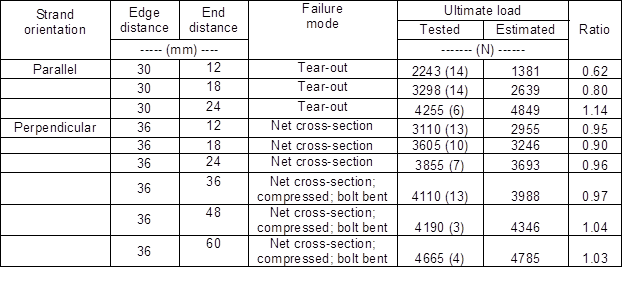The ratio values ranging from 0.62 to 1.14 for connections that failed in end tear-out mode indicates that Eq. 16 estimates mean ultimate lateral resistance loads of connections with their end distance equal or larger than 18 mm, but tends to underestimate mean ultimate lateral resistance loads of connections with their end distance smaller than 18 mm. The ratio values ranging from 0.90 to 1.04 for connections that failed in cross-section tension mode indicates that Eq. 17 overall estimates mean ultimate lateral resistance loads of connections reasonably well, but it still has the trend of underestimating mean ultimate lateral resistance loads of connections with a smaller end distance.

These results indicated that the proposed regression method was a valid experimental approach for obtaining estimation equations of mean ultimate lateral resistance loads of single-shear metal-to-PBSP single-bolt connections evaluated in this study with failure modes of end tear-out and cross-section tension.

To obtain a functional relationship between stress concentration factor, Ks, and end-distance to bolt-diameter ratio, (e/d), of single-shear metal-to-PBSP single-bolt connections that failed in end tear-out mode that might be practical for design purposes, all data points to ultimate lateral resistance loads of the complete 3 × 2 factorial experiment with 4 replications per combination pooled together with mean ultimate lateral resistance load values and their corresponding stress concentration factor values calculated (Table 3). The linear function Eq. 14 was considered to fit average data points by the method of least squares, where a and b are regression constants. The regression analysis yielded the significant least squares Eq. 14,

Ks = 3.791 – 0.362(e/d)

with an R2 value of 0.9997.

For single-shear metal-to-PBSP single-bolt connections failed in cross-section tension mode, all data points of ultimate lateral resistance loads of the complete 6 × 2 factorial experiment with 4 replications per combination pooled together with mean ultimate lateral load values and their corresponding stress concentration factor value calculated (Table 3). The quadratic function Eq. 15, Kt =a (e/d) + (e/d2, was considered to fit average data points by the method of least squares, where ab, and c are regression constants. The regression analysis yielded the significant least squares in Eq. 15,

Kt = – 0.189 + 0.785 (e/d) – 0.031 (e/d2 (15)

with an R2 value of 0.937.

Table 3. Mean Values of Ultimate Lateral Resistance Loads and their Corresponding Stress Concertation Factors of All Single-Shear Metal-to-PBSP Single-Bolt Connections Evaluated in this Study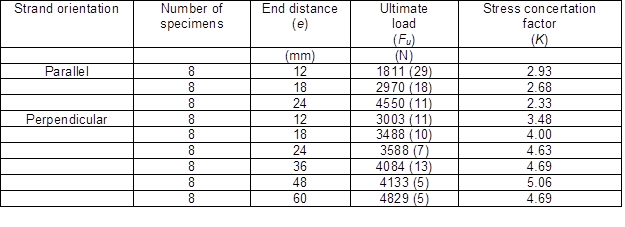a Value in parentheses are coefficients of variation in percentage

Minimum end distance for design consideration

Figure 9 plots all data points of mean ultimate lateral resistance loads of single-shear metal-to-PBSP single-bolt connections versus their corresponding (e/d) values, i.e. including all connections that failed in end tear-out mode when subjected to lateral loads parallel to bamboo strand orientation of main members (indicated by the sloped straight line) and net cross-section tension mode when subjected to lateral loads perpendicular to bamboo stand orientation of main members (indicated by the curvilinear line), and also all connections subjected to lateral loads parallel to bamboo strand orientation of main members and failed in yield failure modes only in main members (indicated by the horizontal line) reported in Yu et al. (2017).

In the case of connections subjected to lateral loads parallel to bamboo strand orientation of main members, the sloped line indicates that the mean ultimate resistance load of a single-shear metal-to-PBSP single-bolt connection increases proportionally to the increase of its (e/d) value. This increase trend in mean ultimate lateral resistance loads can reach its limit, i.e. at the interception between two trend straight lines (Fig. 9), where the (e/d) is 5 and the failure mode of the connection changed from end tear-out to yield failure modes, i.e. both a main member and a bolt in a connection failed in yield modes. This observation indicates that the minimum end distance for a single-shear metal-to-PBSP single-bolt connection evaluated in this study should be equal or greater than 5d, i.e. five times of bolt diameter in order to reach its maximum lateral resistance capacity.